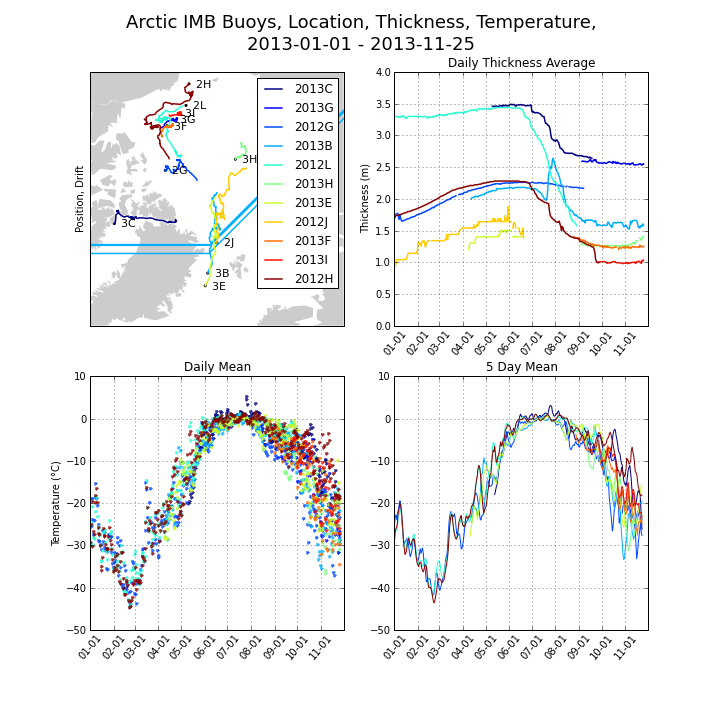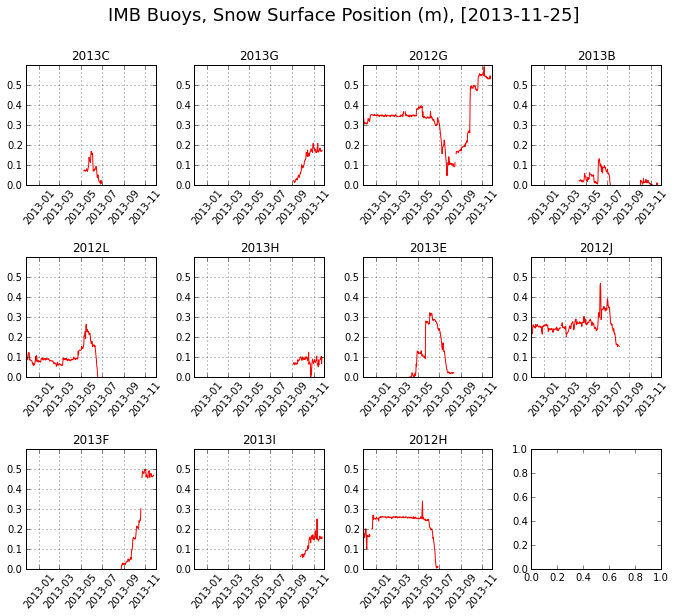# Near realtime data from Arctic ice mass balance buoys

Published: Mon 25 November 2013

Notebook file

Arctic sea ice thickness is a very important information and tells a lot about the state of the floating ice sheet. Unfortunately direct measurements are rare and an area-wide assessment from the ground is too costly. Satellites can fill the gap by using freeboard as a proxy but there are still some obstacles like e.g. determining snow cover. Mass balance buoys offer a near realtime view at a few sites and show the characteristics of melting sea ice in summer and freezing in winter. This notebook accesses latest available data and plots daily thickness, temperature, snow cover and drift of the buoys.

It should be said the data shown here is from a live source and not corrected. The Buoys are not maintained and have to stand forces like strong storms, curious ice bears and hungry foxes. The time lapse video below, shot in 2011, shows the conditions during May to July until the buoy tilted over because the carrying floe melted away.

In :
from IPython.display import YouTubeVideo

Out:

First the needed imports and a version check.

In :
%pylab inline

from IPython.core.display import Image

import os, sys, time, csv, urllib
import numpy as np

from StringIO import StringIO
from datetime import datetime, timedelta
from copy import deepcopy

import matplotlib as mlp
import matplotlib.dates as mdates
import matplotlib.pyplot as plt

from mpl_toolkits.basemap import Basemap

now = datetime.utcnow()

print
print "   now: %s" % now
print "python: %s" % str(sys.version_info[:])
print " numpy: %s" % np.__version__
print "   mpl: %s" % mlp.__version__

Populating the interactive namespace from numpy and matplotlib

now: 2013-11-25 21:10:46.242916
python: (2, 7, 4, 'final', 0)
numpy: 1.7.1
mpl: 1.2.1

WARNING: pylab import has clobbered these variables: ['datetime']
%pylab --no-import-all prevents importing * from pylab and numpy


These helper functions convert raw text data, calculate a running mean, select the last valid numeric data point and the mean of a list.

In :
def floatOrNan(string):
try:
return float(string)
except ValueError:
return np.nan

def runmean(interval, size):
window = np.ones(int(size))/float(size)
return np.convolve(interval, window, 'same')

def lastgood(l) :
for i in range(len(l) -1, -1, -1) :
if not np.isnan(l[i]):
return l[i]
return np.nan

def nm(data, **args):


The buoys names are coded with the year of deployment, an 'A' indicates the first buoy of the year. Interesting for 2013 are:

In :
imbbuoys  = ["2012G", "2012H", "2012J", "2012L"]
imbbuoys += ["2013B", "2013C", "2013E", "2013F", "2013G", "2013H", "2013I"]


This class downloads the data to a local folder, reads available data, calculates daily means und saves the data as a numpy array. Some errors are expectable from a live source. One goal is to deal with them and nevertheless produce an usable result.

In :
class Buoys(object):

data = {}
urlpattern  = "http://imb.crrel.usace.army.mil/irid_data/%s_clean.csv"

## local path, must exist !
localpath = "/home/magik/INTERNET/EARTHPY/earthpy.org/content/notebooks/"
filepattern = localpath + "%s.csv"

def update(self) :
""" downlaod all data files """
counter = 0
print self.localpath + ": saving...",
for b in imbbuoys :
try :
urllib.urlretrieve(self.urlpattern % b, self.filepattern % b)
counter += 1
print b,
except Exception, e :
print "\n", b, str(e), self.urlpattern % b
continue
print "done %s" % counter

return self

""" either reads a single buoy or all """

if buoy is None :
buoys = imbbuoys
else :
buoys = [buoy]

for buoy in buoys :

errors = 0;
counter = 0;
lastDay = 0
dd = [];               ## accumulates data from a single day
self.data[buoy] = []   ## data target

with open(self.filepattern % buoy, 'rb') as csvfile:

try :
date = datetime.strptime(row, "%m/%d/%Y %H:%M")
time = date.strftime("%Y-%m-%d")
day  = date.day

## checks whether this line belongs to a new day
if day <> lastDay and len(dd) > 0 :
## if so, saves the mean of all rows of the day before
dd = np.array(dd)
self.data[buoy].append((
time, nm(dd[:,0]), nm(dd[:,1]), nm(dd[:,2]), nm(dd[:,3]), nm(dd[:,4]), nm(dd[:,5])))

# reset day array
dd = []
lastDay = day

lat  = floatOrNan(row)
lon  = floatOrNan(row)
temp = floatOrNan(row)
pres = floatOrNan(row)
snow = floatOrNan(row)
thck = floatOrNan(row)
dd.append([lat, lon, temp, pres, snow, thck])

except:
errors += 1

finally:
counter += 1

dd = np.array(dd)
self.data[buoy].append((
time, nm(dd[:,0]), nm(dd[:,1]), nm(dd[:,2]), nm(dd[:,3]), nm(dd[:,4]), nm(dd[:,5])))

## did that go successfully ?
print "read %s: %s/%s \t%s : %s" % (
buoy, counter, errors, self.data[buoy], self.data[buoy][-1])

def save(self) :

scheme = [('day', 'datetime64[D]'), ('lat', 'f'), ('lon', 'f'),
('temp', 'f'), ('press', 'f'), ('snow', 'f'), ('thickness', 'f')]

print self.localpath + ": tofile...",

for buoy in imbbuoys :
if self.data.has_key(buoy):
arr = np.rec.array(self.data[buoy], scheme)
arr.tofile(self.localpath + buoy + ".npy")
self.data[buoy] = arr
print buoy,
print " done"

## now run
buoys = Buoys()
buoys.update()
buoys.save()

/home/magik/INTERNET/EARTHPY/earthpy.org/content/notebooks/: saving... 2012G 2012H 2012J 2012L 2013B 2013C 2013E 2013F 2013G 2013H 2013I done 11
read 2012G: 10071/0 	2012-10-01 : 2013-11-25
read 2012H: 10573/0 	2012-09-10 : 2013-11-25
read 2012J: 10378/0 	2012-08-25 : 2013-11-25
read 2012L: 9403/0 	2012-08-27 : 2013-09-25
read 2013B: 5487/0 	2013-04-10 : 2013-11-25
read 2013C: 4787/0 	2013-05-10 : 2013-11-25
read 2013E: 5536/0 	2013-04-08 : 2013-11-25
read 2013F: 2221/0 	2013-08-25 : 2013-11-25
read 2013G: 1964/0 	2013-09-04 : 2013-11-25
read 2013H: 1992/0 	2013-09-03 : 2013-11-25
read 2013I: 1500/0 	2013-09-24 : 2013-11-25
/home/magik/INTERNET/EARTHPY/earthpy.org/content/notebooks/: tofile... 2012G 2012H 2012J 2012L 2013B 2013C 2013E 2013F 2013G 2013H 2013I  done


Before plotting a pre-flight check with the buoys sorted by most recent thickness

In :
bsort = imbbuoys[:]
bsort.sort(key=lambda x: lastgood(buoys.data[x]['thickness']), reverse=True)

print 'Buoy\t', ' -10\t', 'good\t', '  dThk\t', '  Temp\t', '      Last'

for buoy in bsort :
data = buoys.data[buoy]
print buoy, '\t', \
("%.2f" % data['thickness'][-10]).rjust(4), '\t', \
("%.2f" % lastgood(data['thickness'])).rjust(4), '\t', \
("%.3f" % (lastgood(data['thickness']) - data['thickness'][-10])).rjust(6), '\t', \
("%.2f" % data['temp'][-1]).rjust(6), '\t', \
data['day'][-1].astype(datetime).strftime('%Y-%m-%d')

Buoy	 -10	good	  dThk	  Temp	      Last
2013C 	 nan 	2.64 	   nan 	-27.26 	2013-11-25
2013G 	2.54 	2.54 	 0.003 	-29.40 	2013-11-25
2012G 	 nan 	2.17 	   nan 	-28.01 	2013-11-25
2013B 	1.62 	1.58 	-0.042 	-28.39 	2013-11-25
2012L 	 nan 	1.58 	   nan 	 -1.37 	2013-09-25
2013H 	1.34 	1.41 	 0.067 	-29.36 	2013-11-25
2013E 	 nan 	1.40 	   nan 	-20.04 	2013-11-25
2012J 	 nan 	1.39 	   nan 	   nan 	2013-11-25
2013F 	1.24 	1.25 	 0.009 	-34.29 	2013-11-25
2013I 	1.01 	1.03 	 0.027 	-26.96 	2013-11-25
2012H 	 nan 	0.82 	   nan 	-18.68 	2013-11-25


The -10 days thickness column indicates gaps, however all buoys report at least one good thickness measurement. 2012L was decommissioned in late September.

The plotted report card will have a map of the locations of the buoys, a plot of the recorded thickness and two plots of the temperatures. Each buoys is coded with same color in each plot along a rainbow palette, indicating thick ice using blue and thin ice with red.

In :
start = datetime(2013, 1, 1)
ddiff = (now - start).days

fg, axes = plt.subplots(figsize=(10, 10), dpi=72, nrows=2, ncols=2)

ax = axes.flatten()

## decorate the non map plots
for a in ax[1:4] :

a.set_color_cycle([plt.cm.jet(i) for i in np.linspace(0, 0.99, len(imbbuoys))])
a.xaxis.set_major_locator(mdates.MonthLocator(interval=1))
a.xaxis.set_major_formatter(mdates.DateFormatter('%m-%d'))
a.set_xlim(start, now + timedelta(5))
r = [l.set_rotation(50) for l in a.get_xticklabels()]
a.grid(True)

# same color code for the map
ax.set_color_cycle([plt.cm.jet(i) for i in np.linspace(0, 0.99, len(imbbuoys))])

map = Basemap(projection='npstere', ax=ax, resolution='c', area_thresh=5000,
lon_0=0, lat_ts=70, boundinglat=70, rsphere=(6378137.00, 6356752.3142))

map.fillcontinents(ax=ax, color='0.8', lake_color='0.9')

for buoy in bsort :

data = buoys.data[buoy]

lons = data['lon'][-min(ddiff, len(data['lon'])):]
lats = data['lat'][-min(ddiff, len(data['lat'])):]

x, y = map(lons, lats)

# remember last position
lastX = x.compressed()[-1]
lastY = y.compressed()[-1]

# plot map with locations
map.plot(x, y, linewidth=1.5, label=buoy)
map.scatter(lastX, lastY, 4, marker='o', color='black')
ax.text(lastX, lastY, "  " + buoy[-2:], fontsize=11, fontweight='normal', ha='left', va='center')
ax.set_ylabel('Position, Drift')

# thickness
ax.plot(data['day'].astype(object), data['thickness'], linewidth=1.5, label=buoy)
ax.set_ylabel('Thickness (m)')
ax.set_title("Daily Thickness Average");
ax.set_ylim(0, 4)

# Temperature I
ax.plot(data['day'].astype(object), data['temp'], ".", alpha=0.8)
ax.set_ylabel(u'Temperature (°C)')
ax.set_title("Daily Mean");
ax.set_ylim(-50, 10)

# Temperature II
ax.plot(data['day'].astype(object)[3:-3], runmean(data['temp'], 5)[3:-3], alpha=1, linewidth=1)
ax.set_title("5 Day Mean");
ax.set_ylim(-50, 10)

# decoration
lg = ax.legend(loc=1)
pt = plt.suptitle(u'Arctic IMB Buoys, Location, Thickness, Temperature, \n%s - %s' %
(start.strftime("%Y-%m-%d"), now.strftime("%Y-%m-%d")), fontsize=18)

# save image and display inline
imgFile = buoys.localpath + "IMB-Buoys-%s.png" % now.strftime("%Y-%m-%d")
plt.savefig(buoys.localpath + "buoys-plotted.png", dpi=72)
plt.close(fg)
Image(filename=buoys.localpath + "buoys-plotted.png")

Out:Buoy 2013B seems to have some poblems with its GPS device. But the overall results represent an interesting summer. 2012G registered almost no thinnning north of the Canadian Arctic Archipelago and 2012L drifting near the ice edge in the Beaufort Sea saw a loss of 2m in about 3 month.

Last but not least the snow cover overview, plotted straight forward.

In :
fg, axes = plt.subplots(figsize=(9, 10), dpi=72, nrows=3, ncols=4)

for buoy, ax in zip(bsort, axes.flatten()) :

data = buoys.data[buoy]

ax.xaxis.set_major_locator(mdates.MonthLocator(interval=2))
ax.xaxis.set_major_formatter(mdates.DateFormatter('%Y-%m'))

ax.plot(data['day'].astype(object), data['snow'], 'red')
r = [l.set_rotation(50) for l in ax.get_xticklabels()]
ax.set_title(buoy);
ax.set_ylim(0, 0.6)
ax.set_xlim(now - timedelta(365), now + timedelta(5))
ax.grid(True)

plt.subplots_adjust(left=0.01, right=0.99, top=0.90, bottom=0.2, wspace=0.3, hspace=0.6)
pt = plt.suptitle('IMB Buoys, Snow Surface Position (m), [%s]' % now.strftime("%Y-%m-%d"), fontsize=18)